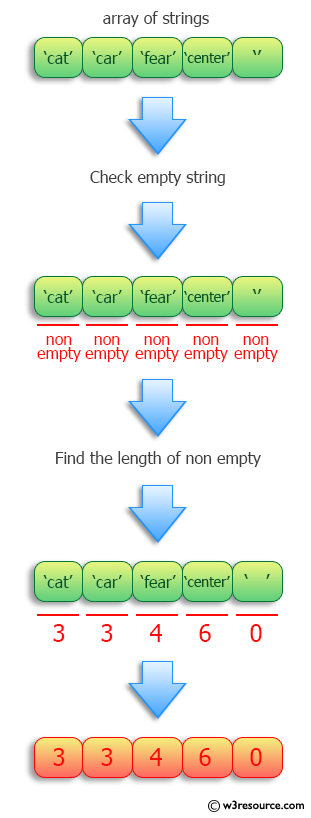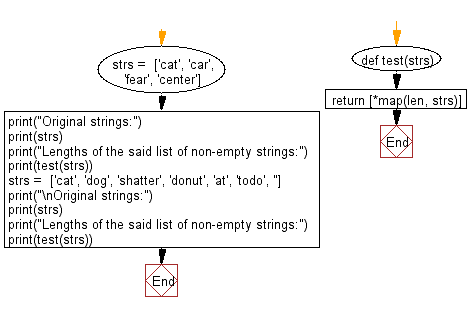﻿ Python: Find the lengths of a list of non-empty strings - w3resource# Python: Find the lengths of a list of non-empty strings

## Python Programming Puzzles: Exercise-14 with Solution

Write a Python program to find the lengths of a given list of non-empty strings.

```Input:
['cat', 'car', 'fear', 'center']
Output:
[3, 3, 4, 6]

Input:
['cat', 'dog', 'shatter', 'donut', 'at', 'todo', '']
Output:
[3, 3, 7, 5, 2, 4, 0]
```

Pictorial Presentation:Sample Solution:

Python Code:

``````#License: https://bit.ly/3oLErEI
def test(strs):
return [*map(len, strs)]
strs =  ['cat', 'car', 'fear', 'center']
print("Original strings:")
print(strs)
print("Lengths of the said list of non-empty strings:")
print(test(strs))
strs =  ['cat', 'dog', 'shatter', 'donut', 'at', 'todo', '']
print("\nOriginal strings:")
print(strs)
print("Lengths of the said list of non-empty strings:")
print(test(strs))
``````

Sample Output:

```Original strings:
['cat', 'car', 'fear', 'center']
Lengths of the said list of non-empty strings:
[3, 3, 4, 6]

Original strings:
['cat', 'dog', 'shatter', 'donut', 'at', 'todo', '']
Lengths of the said list of non-empty strings:
[3, 3, 7, 5, 2, 4, 0]
```

Flowchart:## Visualize Python code execution:

The following tool visualize what the computer is doing step-by-step as it executes the said program:

Python Code Editor :

Have another way to solve this solution? Contribute your code (and comments) through Disqus.

What is the difficulty level of this exercise?

Test your Programming skills with w3resource's quiz.

﻿

## Python: Tips of the Day

Clamps num within the inclusive range specified by the boundary values x and y:

Example:

```def tips_clamp_num(num,x,y):
return max(min(num, max(x, y)), min(x, y))
print(tips_clamp_num(2, 4, 6))
print(tips_clamp_num(1, -1, -6))
```

Output:

```4
-1
```